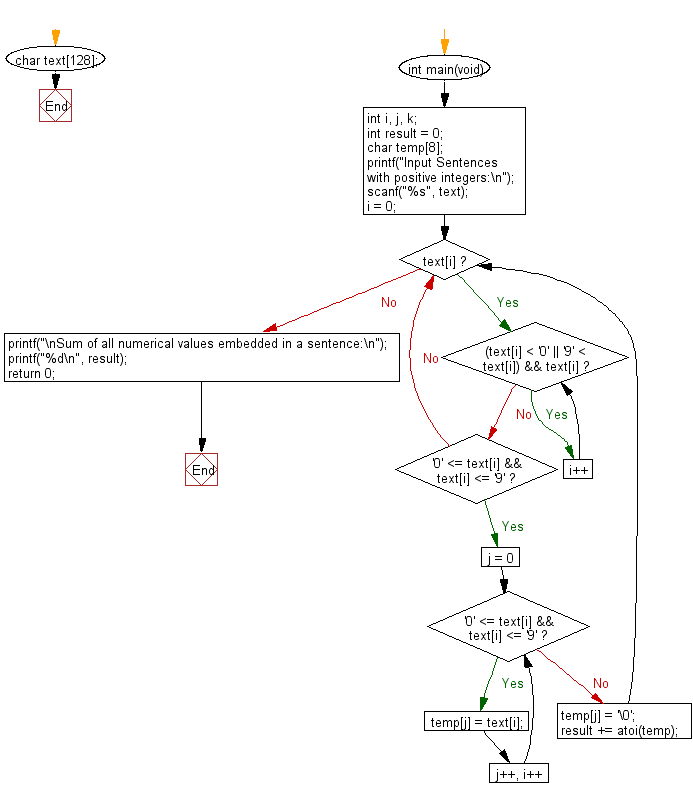﻿ C : Sum of all positive numeric values embedded in a string

# C Exercises: Sum of all numerical values (positive integers) embedded in a sentence

## C Basic Declarations and Expressions: Exercise-145 with Solution

Write a C program to sum all numerical values (positive integers) embedded in a sentence.

Input:
Sentences with positive integers are given over multiple lines. Each line is a character string containing one-byte alphanumeric characters, symbols, spaces, or an empty line. However the input is 80 characters or less per line and the sum is 10,000 or less

Sample Solution:

C Code:

``````#include <stdio.h>
#include <stdlib.h>

// Define a character array to store text
char text;

int main(void) {
int i, j, k;
int result = 0;
char temp;

// Prompt user to input sentences with positive integers
printf("Input Sentences with positive integers:\n");

// Read user input into the 'text' array
scanf("%s", text);

i = 0;
while (text[i]) {
// Loop through characters until a digit is found
for (; (text[i] < '0' || '9' < text[i]) && text[i]; i++);

if ('0' <= text[i] && text[i] <= '9') {
// Extract and store the numerical value in 'temp'
for (j = 0; '0' <= text[i] && text[i] <= '9'; j++, i++) {
temp[j] = text[i];
}

temp[j] = '\0';
// Convert 'temp' to an integer and add it to 'result'
result += atoi(temp);
}
}

// Print the sum of all numerical values embedded in the sentence
printf("\nSum of all numerical values embedded in a sentence:\n");
printf("%d\n", result);

return 0;
}
``````

Sample Output:

```Input Sentences with positive integers:
5littleJackand2mouse.

Sum of all numerical values embedded in a sentence:
7
```

Flowchart:C programming Code Editor:

What is the difficulty level of this exercise?

Test your Programming skills with w3resource's quiz.

﻿